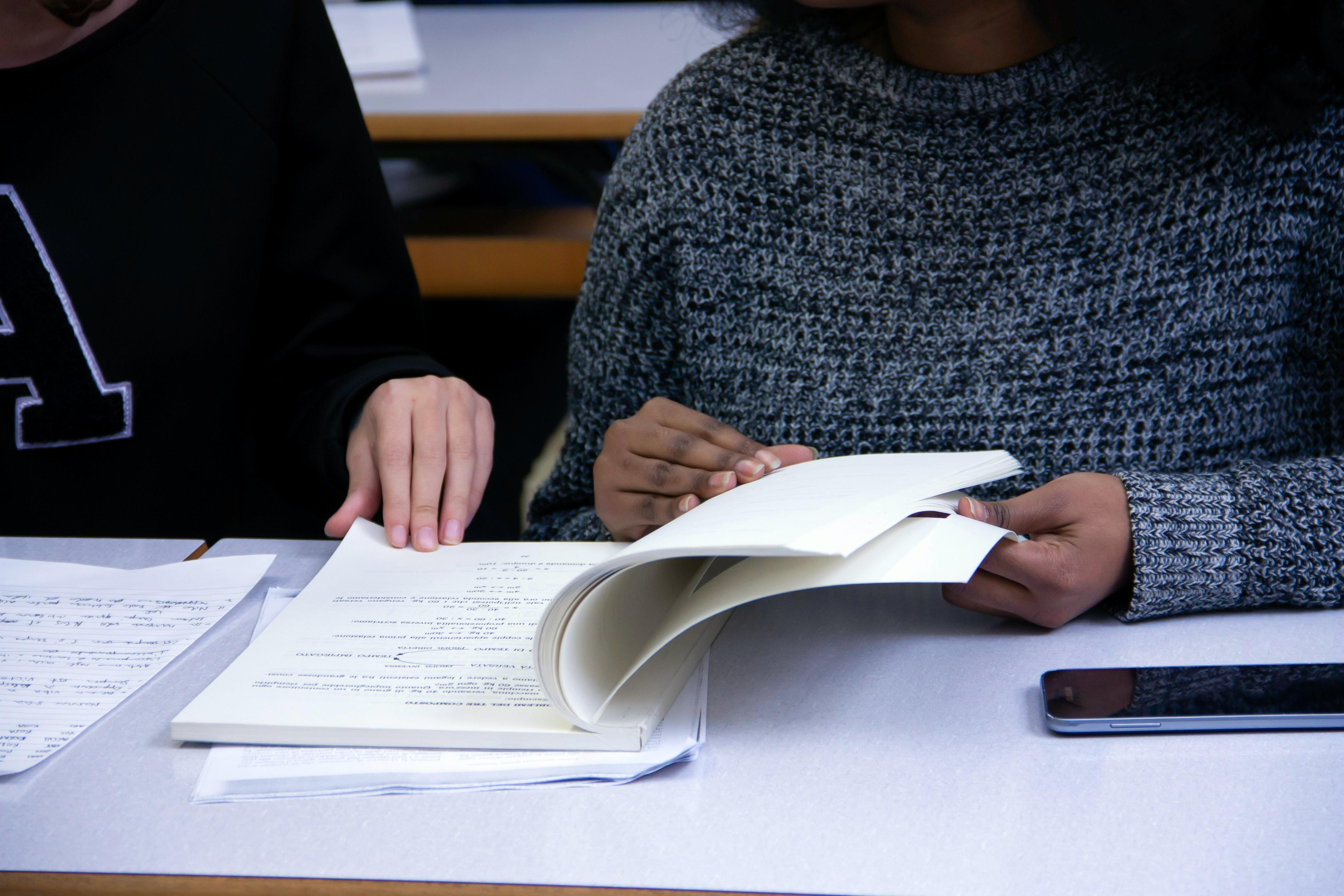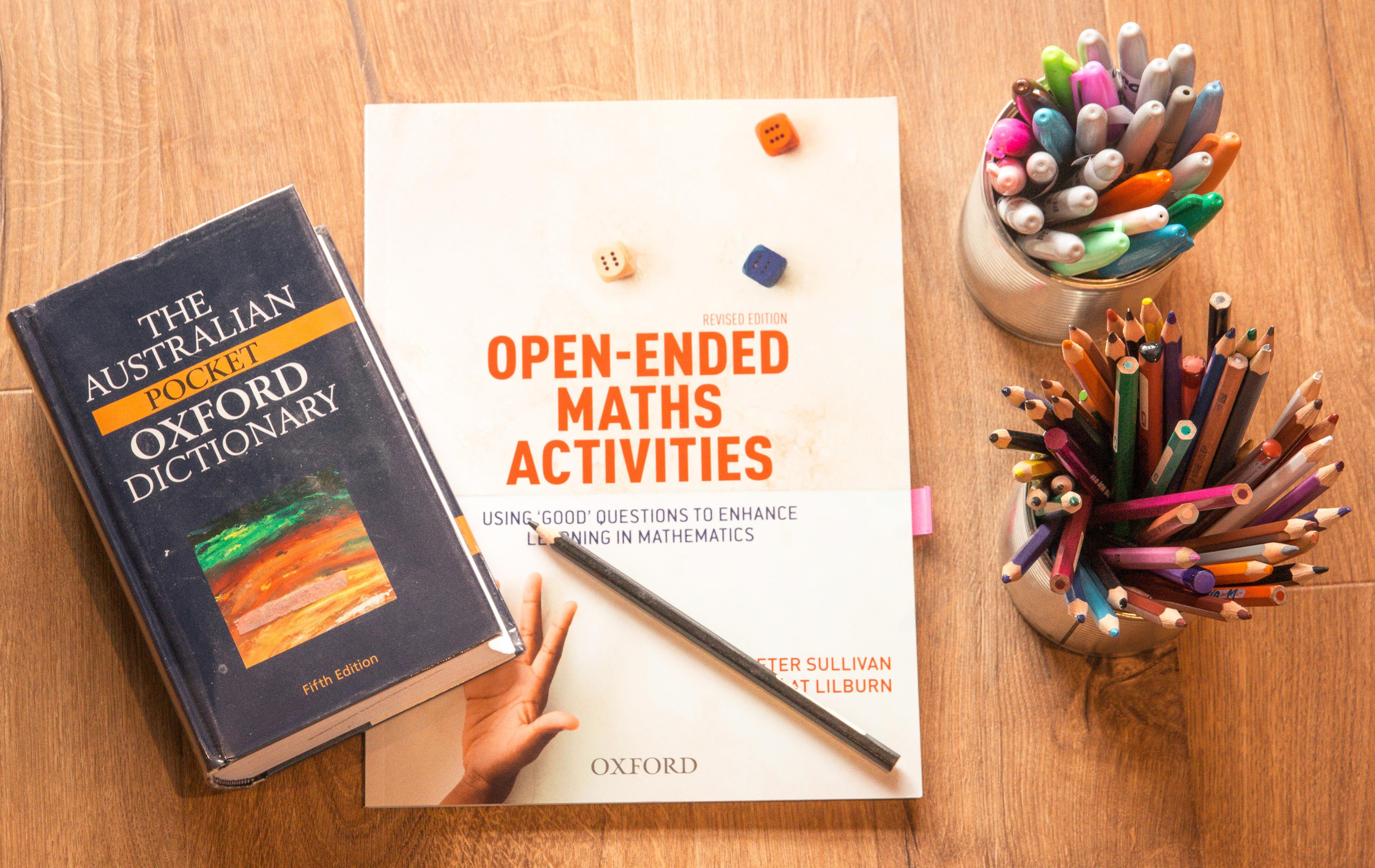"Mathematics is not about numbers, equations, computations, or algorithms: it is about understanding." -William Paul Thurston

Do the mathematical equations and countless numbers turn you away from developing a liking of mathematics? If so, you're not alone in your mindset. The complex equations, numbers, and algorithms have made maths one of the most complicated academic disciplines studied at a primary or secondary school level.

Nonetheless, it is essential to state that maths teachers who have students follow the pages of educational textbooks do not want to torture them. Instead, they recognise that maths is an important topic that must be studied to develop a more thorough knowledge of logical things in life.

The process of studying maths isn't about calculus or algebra. However, those are indispensable topics, but valuable skills such as problem-solving, analytical thinking, logical reasoning, and precise communication make more well-rounded individuals in a community.

So, if you're a secondary school student in Ireland struggling to understand why geometry or probability is needed in your life, try to focus on the positive abilities that you will hone; you have no choice but to view the bright side since maths is a mandatory topic.

Without further delay, we shall analyse how the leaving cert maths curriculum and exam are structured and the mathematical topics that are taught to students.The best Maths tutors available5 (16 reviews)
Mert
€15
/h1st lesson free!4.9 (10 reviews)
Moses
€20
/h1st lesson free!5 (15 reviews)
Khobaib
€14
/h1st lesson free!5 (7 reviews)
Sean
€40
/h1st lesson free!4.8 (6 reviews)
Nirmit
€15
/h1st lesson free!5 (64 reviews)
Dr. Mary (highly experienced teacher)
€45
/h1st lesson free!5 (2 reviews)
Anna
€25
/h1st lesson free!5 (10 reviews)
Omolara
€30
/h1st lesson free!5 (16 reviews)
Mert
€15
/h1st lesson free!4.9 (10 reviews)
Moses
€20
/h1st lesson free!5 (15 reviews)
Khobaib
€14
/h1st lesson free!5 (7 reviews)
Sean
€40
/h1st lesson free!4.8 (6 reviews)
Nirmit
€15
/h1st lesson free!5 (64 reviews)
Dr. Mary (highly experienced teacher)
€45
/h1st lesson free!5 (2 reviews)
Anna
€25
/h1st lesson free!5 (10 reviews)
Omolara
€30
/h1st lesson free!

## How the Leaving Cert Maths Exam and Curriculum is StructuredBy knowing more about Ireland's leaving cert maths exam structure, students can better prepare for their upcoming assessment day. (Source: Unsplash)

By knowing a little more about how the leaving cert maths curriculum is organised and how the end of year examination is structured, students may better understand what to expect shortly.

First and foremost, let's take about the curriculum. The Irish Leaving Certificate is the final exam of the Irish secondary school system that takes a minimum of two years of preparation/study to complete. Students undertaking the leaving cert qualifications are between the ages of 16-20.

The leaving cert prepares students for university classes and gives them the qualifications they need to be deemed worthy of attending post-secondary studies.

While there are many academic disciplines to choose from, the core subjects of study include Irish, English, maths, and European languages. It is essential to mention that students are classified into three levels: higher, ordinary, or foundation in all subjects. The most commonly selected levels are either higher or ordinary.

But, the question may arise, what is examined in the maths section of the leaving cert syllabus? Such as? It is worth mentioning that the leaving cert maths curriculum established by the government entities consists of five strands of study. The following are the five strands of the study examined by students during the maths leaving cert:

1.  Statistics and Probability,
2. Geometry and Trigonometry,
3. Number,
4. Algebra,
5. Functions.

We'll take a further look into the specific topics from each of these five strands in our following subheading.

After the five strands have been studied and carefully looked at across two to three years of study, the leaving cert maths examination must be completed before a diploma is granted. The leaving cert maths test slightly changes the questions year after year; however, the structure remains the same.

The examination is divided into two sections: Paper 1 and Paper 2. Both papers combined take two and a half hours to finish. Paper 1 has a section A and a section B; the same is true about Paper 2. Based on data from past papers, there are approximately six questions for section A and three questions for section B in both papers.

Section A is focused on concepts and skills, and section B primarily directs its attention to contexts and applications.

What is the content in each question? Keep on reading to find out more about the topics from the leaving cert maths exam/curriculum.

## The Mathematical Topics Included in Leaving Cert MathsWorking on maths activities that aren't in textbooks from the teacher will complement the learning experience. (Source: Unsplash)

Based on the curriculum released by Irish education experts, the five strands of maths are considered extensively throughout the two years of studying the leaving cert.

Let's take a further look at the five strands of mathematical content included in the syllabus of the leaving cert and the subdisciplines studied in these branches of maths.

### Algebra

Algebra is one of the broadest terms in mathematics, yet it can be described as studying mathematical symbols and the rules for manipulating these symbols. During the secondary school years, many students seem to have difficulty navigating through all aspects of algebra; however, it is possible to like algebra and view it as efficient and logical.

During the leaving cert maths curriculum and exam, you will experience many questions that are related to the topic of algebra. The following are a few of the algebra concepts, methods, and subdisciplines that a student should recognise:

• Multiplying Brackets,
• Algebraic Fractions,
• Factorisation,
• Simplifying Algebraic Fractions,
• Manipulating Formulas,
• Solving Simultaneous Equations,
• Solving Quadratic Equations,
• Roots of Quadratics,
• Simultaneous Equations, Linear & Quadratic,
• Surds,
• Algebraic Long Division,
• Factor Theorem,
• Polynomials,
• Solving Cubic Equations,
• Verifying Roots in Cubic Equations,
• Finding Unknown Values,
• Completing the Square,
• Number Line,
• Linear Inequalities,
• Modulus.

We remarkably suggest paying attention, taking notes, and asking questions during class when any of the previously mentioned aspects of algebra are brought up since there will 100% be questions that refer to these topics on the final exam.

### Functions

In layman's terms, functions are mathematical entities that assign unique outputs to given inputs. During the leaving cert maths, students take a look at various aspects of functions, including the following:

• Graph cubic, exponential, logarithmic, and trigonometric functions,
• Injective, Surjective, and Bijective Functions,
• Limits and continuity of operations.

Also, it is worth stating that under the umbrella subject of functions, many aspects of calculus are examined, such as limits, calculating the slope, differentiation, points of inflexion, and turning points.

We recommend taking the time to analyse two primary aspects of calculus: differentiation and integration.

Take a look at ExamLearn's notes and tips about studying for the broad branch of functions.Sometimes it may seem that there are so many maths topics that your head might explode. Take your time and study maths topics one by one. (Source: Unsplash)

### Numbers

In the number strand of the leaving cert maths curriculum, examiners and assessors strive to have students continue to make meaning of addition, subtraction, multiplication, and division operations while also trying to understand complex numbers.

In this section of number, students are taught the following topics:

• Number systems,
• Indices,
• Arithmetic,
• Length, area, and volume.

For example, on a past leaving cert maths exams, in the Paper 2 section of the assessment, students were faced with mathematical problems that had to do with length, area, volume, polygons, nets, composite shape problems, etc. trapezoidal rule.

During the number section, the purpose is to expand on your knowledge of calculating complex numbers.

### Geometry and Trigonometry

The next strand of study that has two significant subgenres of maths is geometry and trigonometry. Although not 100% similar in all aspects, both geometry and trigonometry have to do with shapes.

For the geometry section of this strand, the following aspects are analysed throughout the two years of study to complete the leaving cert maths part:

• Lines & Angles,
• Triangles,
• Congruent Triangles,
• Parallelograms,
• Ratio & Parallel Lines,
• Similar Triangles,
• Circles,
• Transformations,
• Enlargements.

Important subdisciplines of geometry such as synthetic geometry, coordinate geometry, and transformation geometry are also analysed by students.

What about trigonometry? As for trig, all the fundamentals of the subject are reviewed to give learners a solid foundation. We recommend taking the time to review the following aspects of trigonometry:

• Sin, Cos & Tan,
• Sine Rule,
• Cosine Rule,
• Uses of Radians,
• Graphs of Sin, Cos & Tan,
• Unit Circle,
• Trigonometric Identities,
• Pythagoras.

### Statistics and Probability

Last but not least, we will discuss what has to do with the ever-important section of statistics and probability.

In this section of the leaving cert maths syllabus, students learn more about the following topics:

• Finding, collecting, and organising data,
• Representing data graphically and numerically,
• Analysing, interpreting, and drawing conclusions from data.

The topic of probability is made easier to understand for students by observing Venn diagrams, arranging, and using the box method.

As for statistics, the following aspects should be well learnt by students to succeed during the final examination:

• Sampling,
• Pie Charts,
• Bar Charts,
• Stem & Leaf Plots,
• Histograms,
• Scatter Plots,
• Line of Best Fit,
• Mean, Median & Mode,
• Frequency Distribution Table,
• Range & Interquartile Range,
• Standard Deviation,
• Percentiles,
• Central Limit Theorem,
• Population Proportion.

In conclusion, before embarking on the maths subject of the leaving cert in Ireland, we highly recommend students to look at this article to know which main branches of mathematical studies are taught to students and which specific topics might be on the final exam.

Preparation is one of the fundamental keys to success. Knowing ahead of time what to expect in the maths curriculum relieves anxiety when studying the important topic of maths!The platform that connects tutors and students5.00 (1 rating(s))Loading...